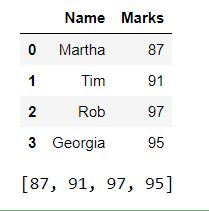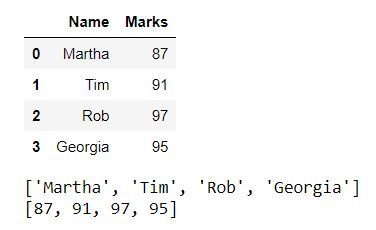Related Articles
Get a list of a particular column values of a Pandas DataFrame
• Last Updated : 28 Jul, 2020

In this article, we’ll see how to get all values of a column in a pandas dataframe in the form of a list. This can be very useful in many situations, suppose we have to get marks of all the students in a particular subject, get phone numbers of all employees, etc. Let’s see how we can achieve this with the help of some examples.

Example 1: We can have all values of a column in a list, by using the tolist() method.

Syntax: Series.tolist().

Return type: Converted series into List.

Code:

## Python3

 `# import pandas libraey ` `import` `pandas as pd ` ` `  `# dictionary ` `dict` `=` `{``'Name'``: [``'Martha'``, ``'Tim'``, ` `                ``'Rob'``, ``'Georgia'``], ` `         ``'Marks'``: [``87``, ``91``,  ` `                  ``97``, ``95``]} ` ` `  `# create a dataframe object ` `df ``=` `pd.DataFrame(``dict``) ` ` `  `# show the dataframe ` `print``(df) ` ` `  `# list of values of 'Marks' column ` `marks_list ``=` `df[``'Marks'``].tolist() ` ` `  `# show the list ` `print``(marks_list)`

Output:Example 2: We’ll see how we can get the values of all columns in separate lists.

Code:

## Python3

 `# import pandas library ` `import` `pandas as pd ` ` `  `# dictionary ` `dict` `=` `{``'Name'``: [``'Martha'``, ``'Tim'``,  ` `                ``'Rob'``, ``'Georgia'``], ` `        ``'Marks'``: [``87``, ``91``,  ` `                 ``97``, ``95``]} ` ` `  `# create a dataframe object ` `df ``=` `pd.DataFrame(``dict``) ` ` `  `# show the dataframe ` `print``(df) ` ` `  `# iterating over and calling  ` `# tolist() method for  ` `# each column ` `for` `i ``in` `list``(df): ` `   `  `    ``# show the list of values   ` `    ``print``(df[i].tolist()) `

Output:Attention geek! Strengthen your foundations with the Python Programming Foundation Course and learn the basics.

To begin with, your interview preparations Enhance your Data Structures concepts with the Python DS Course.

My Personal Notes arrow_drop_up
Recommended Articles
Page :